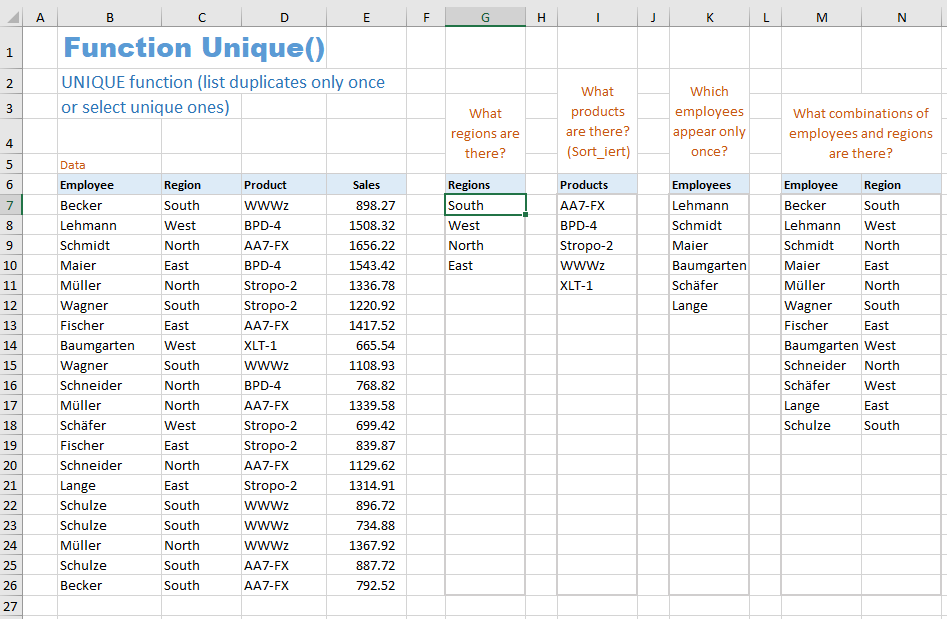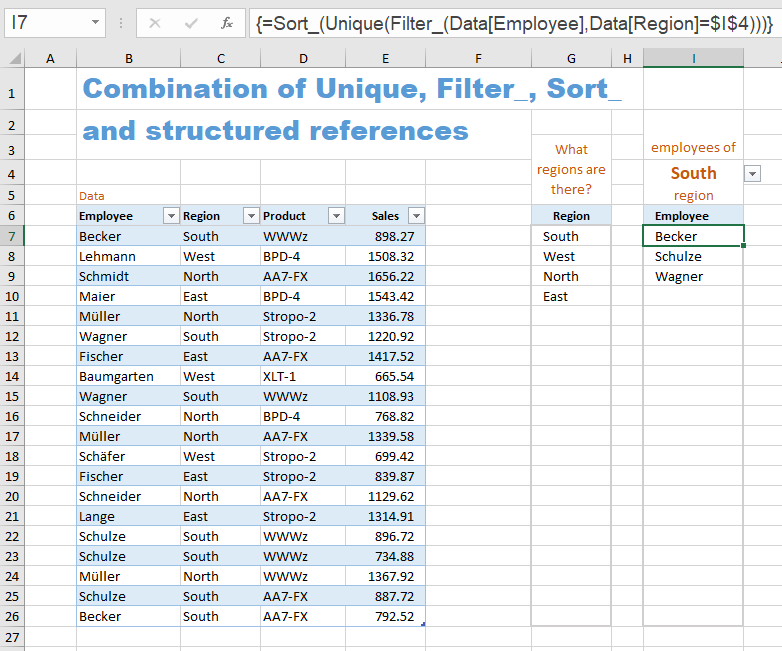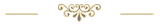Last update: 08.06.2022Download the Excel file with the VBA code of the functions SORT, SORTBY, FILTER, XLOOKUP, XMATCH, UNIQUE, SEQUENCE, RANDARRAY and all examples shown on these web pages:
(Version 3.4 - Last update: 2022-03-15)

In Excel 365 and from Excel 2021, Microsoft implemented the long-missing cell functions SORT, SORTBY, FILTER, XLOOKUP and others. However, they were not retrofitted for older versions of Excel, so that all users from Excel 2007 up to and including Excel 2019 had to do without them.

There is now a solution for users of older versions of Excel (since the end of January 2022).

With the help of VBA so-called UDFs (user defined functions) were implemented that have exactly the same parameter lists as the corresponding Microsoft functions.

In cases where the functions provided here return multiple values, their input must be terminated with CTRL-SHIFT-ENTER. Before entering the formula, an area must be selected on the spreadsheet that contains the returned data - as is usual with the so-called CSE array formulas (CSE = Control Shift Enter).

This tutorial provides a basic and detailed introduction to array formulas.

With Function names of UDFs it doesn't matter whether the letters are written in upper or lower case.

For instructions on intergrating the functions in your own Excel file, see ▸Integrating the functions.

### UNIQUE function

The Unique function is a replacement for the UNIQUE function which is only available in Excel 365. It expects exactly the same parameter list as the Excel 365 function.

= Unique ( array, [by_columns], [exactly_once] )

parameter explanation
array The range or array from which to return unique rows or columns
by_columns
(optional)
A Boolean value that indicates whether to compare rows or columns
TRUE:  Columns are compared and returned
FALSE: Rows are compared and returned
(default)
exactly_once
(optional)
A Boolean value
TRUE:  All rows or columns that occur exactly once are returned
FALSE: The duplicates are removed from the rows or columns
(default)

https://support.microsoft.com/en-us/office/unique-function-c5ab87fd-30a3-4ce9-9d1a-40204fb85e1e

Depending on the value of the third parameter, this function fulfills two different tasks. If the third parameter is not specified or FALSE, all duplicates are removed from the rows/columns and each row/column is listed only once. If the third parameter is TRUE, all rows/columns that occur exactly once are selected. Rows/columns that occur more than once are thus completely omitted.

Matching two rows/columns means that all cells of the first row/column match the corresponding cells of the second row/column.

#### Examples of Unique

Here are some examples for using the Unique function, in the second example also the combination with Sort_.The formulas used are:

= Unique (\$C\$7:\$C\$26)
= Sort_ (Unique (\$D\$7:\$D\$26))
= Unique (\$B\$7:\$B\$26, , WAHR)
= Unique (\$B\$7:\$C\$26)

#### Example for the combination of Sort_, Filter_ and Unique

This example shows how to list all employees of region "South" in alphabetical order.With the formula expression
Filter_ (Data[Employee], Data[Region]=\$I\$4),
all employees of the "South" region are first filtered out (in the drop-down selection field I4 the "South" region should be selected).
To ensure that no employee is listed more than once, the entire formula expression is inserted into the Unique function:
Unique (Filter_ (Tabelle[Mitarbeiter], Tabelle[Region]=\$I\$4)).
In order to sort the employee list, this second formula expression is used in the Sort_ function:
= Sort_ (Unique (Filter_ (Tabelle[Mitarbeiter], Tabelle[Region]=\$I\$4))).Sharing knowledge is the future of mankind